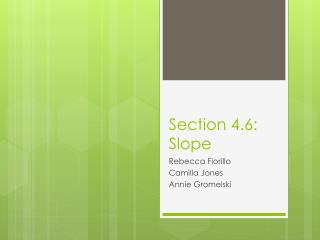Download PresentationSection 4.6: Slope# Section 4.6: Slope - PowerPoint PPT Presentation

Download Presentation##### Section 4.6: Slope

Download Policy: Content on the Website is provided to you AS IS for your information and personal use and may not be sold / licensed / shared on other websites without getting consent from its author. While downloading, if for some reason you are not able to download a presentation, the publisher may have deleted the file from their server.

- - - - - - - - - - - - - - - - - - - - - - - - - - - E N D - - - - - - - - - - - - - - - - - - - - - - - - - - -
##### Presentation Transcript

1. Section 4.6: Slope Rebecca Fiorillo Camilla Jones Annie Gromelski

2. What is slope? • Slope equals Rise/Run. • The slope of non-vertical line, segment, or ray containing (x1,y1) and (x2,y2) is defined by the formula m=y2-y1 or m=y1-y2 x2-x1 x1-x2

3. What is slope? Continued • Slope is used to find out or to describe the measurement of the steepness, incline, gradient, or grade of a straight line. Two points are used to find the slope with the y-points (rise)divided by the x-points (run). • In other words, you are finding the ratio of the altitude change to the horizontal distance between any two points on the line.

4. Slope of Parallel and Perpendicular Lines • Theorem 26: if two non-vertical lines are parallel, then their slopes are equal. Example: If RF ll AG and mRF =2/5 then mAG=2/5 • Theorem 27: if the slopes of two non-vertical lines are equal, the lines are parallel. Example: if mCJ=3/7 and mMP=3/7, then the lines are parallel.

5. Visual interpretations of slope Positive slope negative slope Zero slope no slope

6. Sample Problems mAB=y2-y1 x2-x1 mAB= 5-(-3) 4-(-2) B (4,5) mAB= 8 = 4 6 3 A (-2, -3)

7. Sample Problems Are these lines parallel? A (1,6) C (5,6) The Slopes are not parallel . mAB=6-1 = 5The lines are not opposite 1-8 -7 reciprocals due to the fact that CD is a horizontal line mCD=6-0 = 6which has no slope. 5-5 0 D (5,0) B (8,1)

8. Sample Problems Are these lines parallel? C (2,10) A(2,6) mAB=6-2 =4 =2 = -2 2-4 -2 -1 mCD=10-4 =6 = 3 2-6 -4 -2 B(4,2) D(6,4) The lines are not parallel because they do not have the same slope.

9. Example Problems Given: D is the midpoint AC B( 13,12) Find : 1. Slope of AC A (3,6) 2. Slope of BD DF C(9,2)

10. Answers • -2/3 • 8/7

11. Example Problem 1.Given the points A=(3,5) and B=(4,9). Find the slope of line AB. 2. What is the slope of a horizontal line?

12. Answers • mAB= 4 • The slope of a horizontal line is 0.

13. Example Problems • Find slope of AC • Find slope of AB C(6,6) A(4,-4) B(8,-10) 3. Find slope of CB

14. Answers • mAC = 5 • mAB = 3/-2 • mCB = -8

15. Works Cited "Math Facts.info: Slope." Math Facts.info: Mathfacts. Web. 19 Jan. 2011. <http://mathfacts.info/slope.html>.